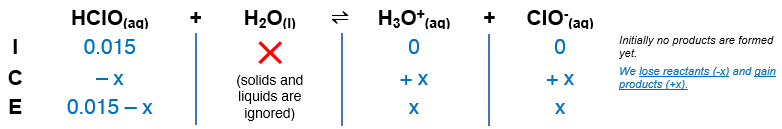# Problem: The Ka of hypochlorous acid (HClO) is 3.0 x 10-8 at 25.0 °C. What is the percent ionization of hypochlorous acid in a 0.015 M aqueous solution of HClO at 25.0°C?a. 0.14b. 4.5 x 10-8 c. 1.4 x 10-3 d. 14e.  2.1 x 10-5

###### FREE Expert Solution

Remember that weak acids partially dissociate in water and that acids donate H+ to the base (water in this case).

The dissociation of HClO is as follows:

HClO(aq) + H2O(l)  H3O+(aq) + ClO(aq)Ka = 3.0 × 10–8

From this, we can construct an ICE table. Remember that liquids are ignored in the ICE table and Ka expression.The Ka expression for HClO is:

88% (217 ratings)###### Problem Details

The Ka of hypochlorous acid (HClO) is 3.0 x 10-8 at 25.0 °C. What is the percent ionization of hypochlorous acid in a 0.015 M aqueous solution of HClO at 25.0°C?

a. 0.14
b. 4.5 x 10-8
c. 1.4 x 10-3
d. 14
e.  2.1 x 10-5